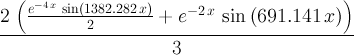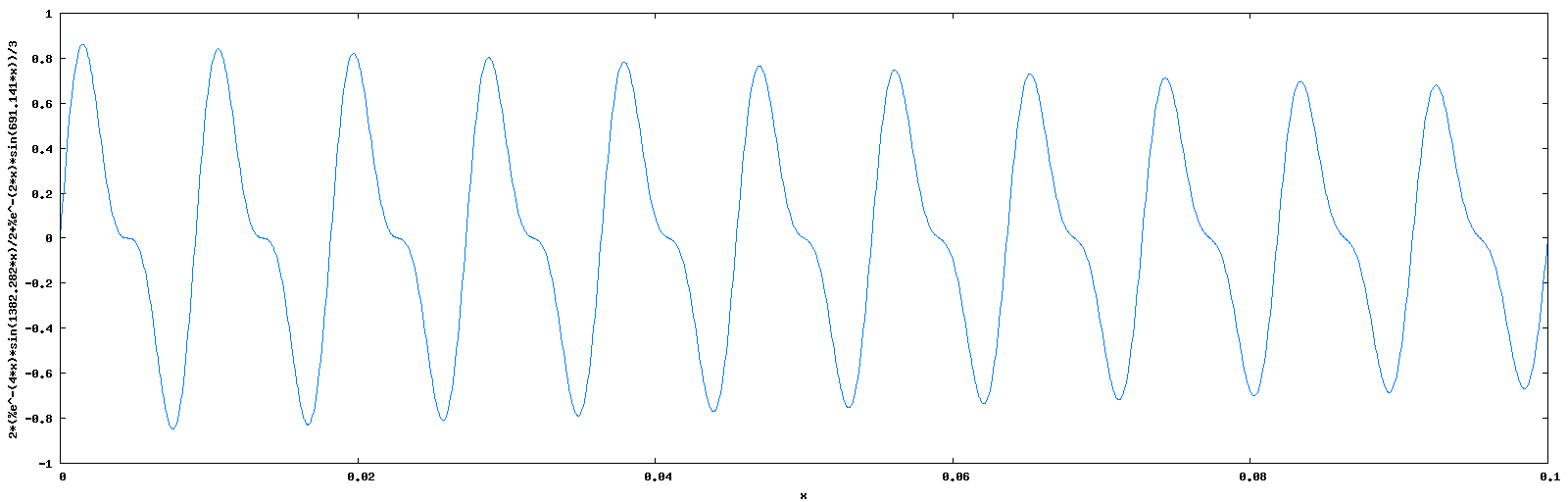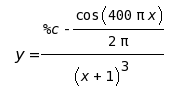### Theo Verelst Mathematical Waves Main Page

After some thinging I decided to make a good web application to automatically create audio wave files from amthematical formulas.

Why? Because that is fun, instructive, complicated a challenge and it promotes what I want, and it is also rather handy to try things out in audioland.

In essence I let a user with a browser make an algebraic formula, or anyone can click on a pre-cooked one, which is fed in sequence to Maxima, a fortran/C program which is compiled with gcc, and then leads to a program which is executed on this server to generate a wav file of a few seconds, which represents the formula in audio form! TO reduce download speed this result is even automatically turned into a mp3 sound file you can download.

Really, that all will happen automatically for in principle any correct Maxima type formula which you feed to the cgi script I made. You may want to test it out, so here are some examples:

sin(440*2*3.1415*x) a 440 Hz continuous sine wave

( sin(440*2*3.1415*x) + sin(2*440*2*3.1415*x) ) /2 a 440 Hz continuous sine wave and it's second harmonic

sin(220*2*3.1415*x)/(1+10*x) a 220 Hz sine wave damped by a fractiona 220 Hz sine wave and it's second harmonic both (differently) exponentially damped
Result looks like this click to image to see this 'live':```(
sin(  6.2831*110*x)*exp(- 2*x)
+(1/2)*sin(2*6.2831*110*x)*exp(- 4*x)
+(1/3)*sin(3*6.2831*110*x)*exp(- 6*x)
+(1/4)*sin(4*6.2831*110*x)*exp(- 8*x)
+(1/5)*sin(5*6.2831*110*x)*exp(-10*x)
+(1/6)*sin(6*6.2831*110*x)*exp(-12*x)
+(1/7)*sin(7*6.2831*110*x)*exp(-14*x)
+(1/8)*sin(8*6.2831*110*x)*exp(-16*x)
+(1/9)*sin(9*6.2831*110*x)*exp(-18*x)
) / (
1+(1/2)+(1/3)+(1/4)+(1/5)+(1/6)+(1/7)+(1/8)+(1/9)
)
```
a complicated set of differently exponentially damped harmonics of a sawtooth wave, slowly becoming more sinusoidial (less overtones)

rhs(ic1(ode2(x^2*'diff(y,x)+3*y*x=sin(10*x)/(10*x),y,x),x=%pi,y=0)) The solution of a 2d order differential equation computed!

rhs(ic1(ode2((x+1)^2*'diff(y,x)+3*y*(x+1)=200*sin((x+1)*2*%pi*200)/(x+1),y,x),x=0,y=0)) The solution of a 2d order differential equation computed, in a form which can be listened to by scaling and time scaling and shifting the solution :Note that you can fill in a formula yourself after the question mark in the cgi page url, which can even be pretty long and complicated, and even contain symbolic integration or differentiation, everything Maxima (see manual) allows you to do with formulas.

Nota Bene ! This is an experimental setup, don't hesitate to comment, try again (slowly, don't reload during the maximum 1/2 minute computations) or experiment. I'm aware of character translation errors, for instance it seems certain browsers don't pass ^ unchanged, and also keep in mind these scripts utilize some heavy programs like maxima, a compiler, latex, and gnuplot and a set of scripts (mainly tcl based) to make the setup a bit internet use -safe, but the server machine in the meanwhile has up to 20,000 hits per month to serve as well, and it doubles as TV (see the Birdcam) and radio and general machine for my use at times and often as webbrowser too, so it can be loaded at times.

Also beware that this whole sequence of processing leads to unique audio files, which are in mono CD quality and there is not even a single ready-made element in these audio results, everything is computed on-the-fly, nothing is pre-sampled or taken from memory.

www.theover.org     Webmaster: Theo Verelst